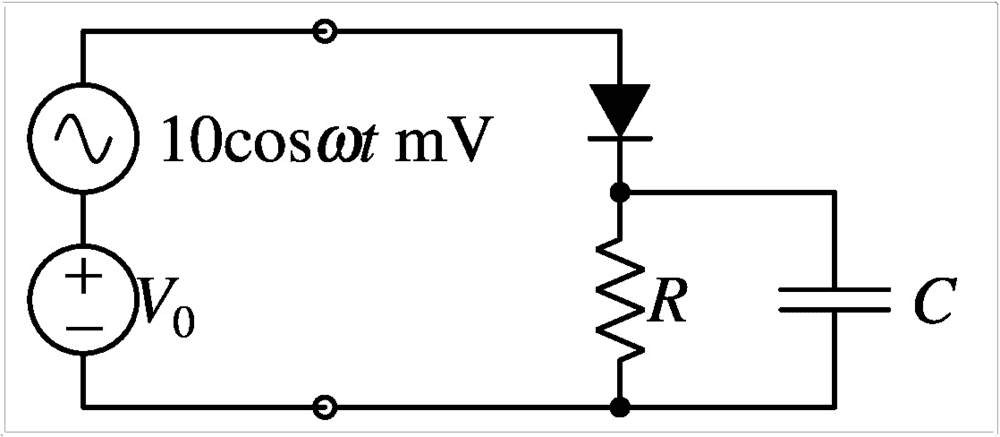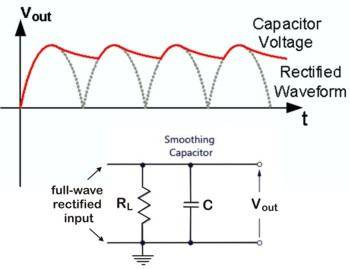# Diode circuit with a Capacitor

• Engineering
Thread moved from the technical forums, so no Template is shown
Summary: Seek help with the working of a Capacitor in the presence of a DC & AC voltage source.

I greatly appreciate this opportunity to submit a question. It's a homework problem and I only seek to clear my concepts.

Problem: Find amplitude of the ripple voltage across the resistor.Concepts: Concepts that I am familiar with are related to the diode. The diode circuit I worked on successfully included just a resistor, and so the ripple voltage was found by determining diode current for the DC source and subtracting from it the diode current for the AC source; the result multipled with R gave me the incremental voltage.

I am unable to proceed with the capacitor in place.

Qualitatively, how does the capacitor behave?

I understand it charges to maximum voltage, which would be the voltage across the resistor. After that, how is its behavior related to alternating voltage?

Best Regards,
wirefree

NascentOxygen
Staff Emeritus
The diode circuit I worked on successfully included just a resistor, and so the ripple voltage was found by determining diode current for the DC source and subtracting from it the diode current for the AC source; the result multipled with R gave me the incremental voltage.
How did you determine the diode current for the AC source in your earlier exercise where there was no capacitor?

DaveE
Gold Member
This circuit is trickier than it might appear, analysis-wise. First, let's look at the extreme cases.
1) Very small capacitor: The circuit will behave as if there is no capacitor, which would be just the positive half of the input sine wave.
2) Very large capacitor: The output voltage will be constant with a value equal to the positive peak of the sign wave input.

In more realistic cases, you will end up with a waveform like this:This is for the full-wave rectifier, but the idea is the same for your half wave rectifier but with zero input for half of the cycles shown. Notice that current only flows during short periods in the low ripple cases, this creates more stress on the circuit components than many people realize.

Unfortunately this is hard to solve analytically. For large capacitors (= light loads, = low ripple), you can approximate this with a piece-wise linear output voltage. It is easy to calculate what is happening when the diodes are off, that is just the capacitor discharging to the load, often that is good enough. In practice, this is a great circuit to simulate to get the answers. I like LTSpice, it's free good, and easy to use. In the dark ages when simulators were not so easy to use we use to have tables and graphs to refer to.

There was an old (late 1970's) National Semiconductor Power Supply design book that had a great section for reference on linear power supply rectifier circuits. I really wish I had kept my copy, but I left it behind when I switched jobs assuming that the content was available on the web, or that I would just use a simulator.

•wirefree
DaveE
Gold Member
Also, I just assumed this was a power supply rectifier application. However, this circuit is very common in analog circuits. It is commonly called a "peak detector" circuit (for obvious reasons) and is very common in RF circuits where it is used to measure the amplitude of RF signals for things like power meters (cheap application specific versions) or in AGC circuits and such.

Many thanks, DaveE, and my apologies to NascentOxygen - the attempt I referred to had only a DC source.

Thanks, again, to DaveE for sharing how a circuit like this is used in practise, i.e. in communication systems. I am hoping to make that field of vocation my bread’n’butter.

A few concerns remain despite relating the problem to rectifier. I will attempt to articulate them in my next post.

Best Regards,
wirefree

NascentOxygen
Staff Emeritus
A few concerns remain despite relating the problem to rectifier. I will attempt to articulate them in my next post.
I don't believe the examples cited by DaveE are anywhere close to the answer your teacher is looking for. Consider operation if the capacitor were not present, like I understood you to say you had previously addressed.

Greatly appreciate your concern, NascentOxygen. I am, indeed, proceeding with basics.

Some concerns I can share at the moment:
• I don't believe diode current changes as a result of 10mV cosine AC signal atop a 1.24V DC source. I am certain.
• In the AC model for a diode, I am considering just the dynamic resistance across the diode as a simplification, which I obtain as a mere 2.6 Ohm. I hoped to consider a drop across this resistance to yield the voltage across the resistor R, which I hoped, again, would yield the needed ripple voltage. But it turns out that for the diode current, as calculated under DC conditions, the drop across the dynamic resistance exceeds the applied AC 10mV! I found that mildly hilarious.
• Before attempting any of the above, I obtained capacitor current. I wonder if my approach was right; I obtained dV/dt as (10mV-0) divided by AC signal time period by 4. I wonder if this is a good shot.

I am well aware that I should be reading up more before posting any more here. Some pointers along the way would be useful.

Best regards,
wirefree

NascentOxygen
Staff Emeritus
AC signal atop a 1.24V DC source
This is the first mention you've made of an actual value. So you have values for R and C, too?

You probably need to calculate the impedance of C in parallel with R.

DaveE
Gold Member
With the presence of both a DC and AC source, the first thing to consider is when, if, how, the diode switches on and off.

Thanks NascentOxygen and DaveE for hanging around.

I am going to take this opporunity to share some numbers, which I avoided to keep things general and not burden you.

Here are some:
- DC at 1.24V
- AC at 10mV cosine
- R at 4.9K Ohm
- C at 5 nF
- Diode Threshold Voltage at 0.65V

Clearly, diode stays on all the time.

Where do I from there?

Best,
wirefree

DaveE
Gold Member
If the diode is always on, you can model it as a battery and a small series resistor. Often we will skip the resistor though, it's usually not that significant.

NascentOxygen
Staff Emeritus
- AC at 10mV cosine
Since the problem specifies a numerical value for every other relevant parameter, I think you have probably been given a value for ω, also?

Many thanks, DaveE. I noted that battery model in Prof. Razavi's (UCLA) text on microelectronics. I am glad to have made a start!

I'd appreciate if you could review my approach thus far:
1. Consider diode is always on
2. Forget DC
3. Convert AC, R and C to phasors
4. Invert R & C phasor values to obtain admittances. Add them. Invert the result.
5. Obtain total current by dividing voltage and impedance phasors from 3 & 4 above
6. Invoke current divider for R to obtain current through R
7. Multiply the last result with R to obtain ripple voltage across R
8. Divide by sqrt(2) to obtain peak value

I tried the above approach but the answer came out wrong. I'll recheck upon receiving your approval of the above approach.

I'd also be indebted if you could share a thought or two about the utility of working with phasors. I've been told they make working with phase angles easier. Sorry, I am new to this.

Best regards,
wirefree

DaveE
Gold Member
OK, some step by step comments:
1) Consider diode is always on
OK, that is a reasonable assumption, I guess. We don't know because you never told us the frequency of the AC source. In any case, you can go ahead with your solution with this assumption, but, you MUST verify that the solution you get is compatible with this. Specifically, you will need to look at the diode current and verify that it doesn't ever flow backwards. If that's true then your assumption was correct. If it isn't true then you need to start over without making this assumption.
2) Forget DC
You can't forget the DC source. However, because you have assumed that the diode is always on and have replaced it with a battery and/or resistor, then you can solve the circuit with the DC source set to zero and also solve it when the AC source is set to zero. Your final solution will be the sum of these 2 separate solutions. For example, the resistor current will be the current due to the DC source plus the current due to the AC source. This only works if your circuit has the basic (linear) circuit elements (resistors, capacitors, voltage sources, etc.), no diodes, switches, or elements with complex behavior. This analysis technique is called superposition and is probably something you haven't studied yet. It is a powerful principle for all "linear" physics problems (it is actually the definition of "linear" in analysis).
3) Convert AC, R and C to phasors
OK. Recall that the magnitude of the capacitors phasor (impedance) is a function of the frequency. That's why we asked about that value.
4) Invert R & C phasor values to obtain admittances. Add them. Invert the result.
Good. That will give you a single impedance value that you can substitute for the R & C parallel combination.
5) Obtain total current by dividing voltage and impedance phasors from 3 & 4 above
OK. The total current is the current through the diode due to the AC source if the diode is always on. This is half of what you need to verify your initial assumption in step 1. You can compare this t the total current from the DC source to determine if the diode current ever flows backwards.
6) Invoke current divider for R to obtain current through R
???
Why do you want to know the current through R?
At step 5 you found the total current from the voltage across R||C. Why wouldn't you use the same approach to find the current through R.
Question: If I put 5V across a 2ohm resistor, what is the current through the resistor? If I then add a 1ohm resistor in parallel with the 2ohm resistor, then what is the current through the 2 ohm resistor.
7) Multiply the last result with R to obtain ripple voltage across R
So you used the voltage across R in step 5 to find the total current, then you calculated the fraction of that current flowing through R, then you multiplied by R to find the voltage across R. Think more, calculate less. You are making the problem unnecessarily hard. However, if you did the calculations correctly, you would get the correct voltage.
8) Divide by sqrt(2) to obtain peak value
This is a typo, right? 1/√2 is about 71%. The peak value is the largest value a quantity can be (in time), which is probably not 71% of something.

OK finally, yes phasors are an important tool. It is important to fully understand the use of phasors (or vectors, or complex numbers, they are all nearly the same thing) in AC circuit analysis. If you continue to study electronics you will have to understand these concepts. They all ultimately come back to working with the magnitude and the phase of sinusoidal waveforms.

Thank you for your detailed feedback, DaveE. I'll be mindful of that when I run through my calculations.